In this post, we will describe the Adaboost classification algorithm. We will start with the basic assumptions and mathematical foundations of this algorithm, and work straight through to an implementation in Python from scratch.

## Motivation: What is a Adaboost Classifier?

In this post, we will describe the Adaboost classification algorithm. We will start with the basic assumptions and mathematical foundations of this algorithm, and work straight through to an implementation in Python from scratch. Adaboost stands for “Adaptive Boosting”, and this was the first boosting technique to gain wide popularity. The algorithm was originally developed by Freund and Schapire 1997.

In a previous post, I introduced the concept of boosting classification and built a simple boosting classifier in Python. I also compared its performance to a single Decision Stump and Random Forest. That model made use of a fixed learning rate during training. The learning rate was used to update the sample weights during each training iteration.

Here we will dispense with the learning rate parameter entirely. Instead, the sample weights will be set dynamically at each training step. This is the reason why the algorithm is termed “adaptive”. In this way, we can build a boosting classifier with significantly better performance. Like all other ensemble techniques, Adaboost combines multiple simple learning models (i.e. “Weak Learners”) into a single powerful classifier. Note that the specific implementation covered in this article follows the Adaboost binary classifier, described in Freund and Schapire 1997.

## Assumptions and Considerations

Some key points to keep in mind when using a Adaboost classifier:

• This algorithm is strictly designed to handle labels with only two unique values
• It is important to make the ensemble of sufficient size to obtain good results
• Assumptions of the weak learner, used to build the ensemble, should be considered. In general, the weak learner should be a very “simple” model
• Since boosting is a sequential algorithm, it can be slow to train. A long training period can affect the scalability of the model

## Derivation of a Adaboost Classification Algorithm

To begin, let’s define the weak learner, loss function, and available data. We will assume there are a total of N samples available in the data, and our ensemble consists of M weak learners. We will index through each unique sample in the data with n = 1..N. Likewise, we will index through each weak learner model, in our ensemble, with m = 1..M.

\bold{X} = \begin{bmatrix} \bold{x}_1 \\ \bold{x}_2 \\ . \\ . \\ \bold{x}_n \\ . \\ \bold{x}_N \end{bmatrix}, \bold{y} = \begin{bmatrix} y_1 \\ y_2 \\ . \\ . \\ y_n \\ . \\ y_N \end{bmatrix}, where \bold{x}_n \in R^{d}, y_n \in \{-1,+1\}    (1)

f_m(\bold{x}_n) \in \{-1,+1\}    (2)

\ell(f_m(\bold{x}_n),y_n) = \{ \begin{array}{rcl} 0 & \text{if} & f_m(\bold{x}_n) = y_n \\ 1 & \text{if} & f_m(\bold{x}_n) \ne y_n \end{array}    (3)

Here equations (1), (2), and (3) describe the data, weak learner model, and loss function, respectively. Note \bold{x}_n are the rows of matrix \bold{X}, and contain d features. Similarly, y_n are the scalar values of the column vector \bold{y}.

With these definitions in place, we can now specify our training procedure. Like all other boosting methods, Adaboost is a sequential algorithm. The weak learners f_m are trained one after the other on the available data (\bold{X},\bold{y}), with the sample weights \bold{w} updated at each step in the sequence. This is done to place more focus on mistakes made during the previous step. A model weight \alpha_m also needs to be assigned to the mth weak learner, in order to properly combine it with the rest of the ensemble. We can illustrate this procedure below, for a small ensemble consisting of M = 4 weak learners: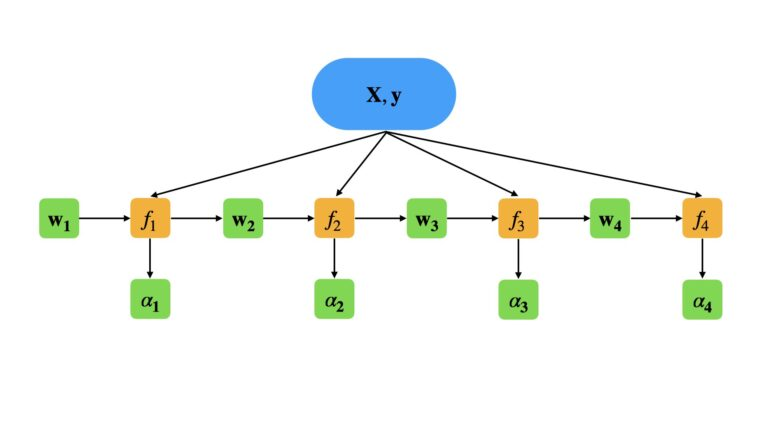The specific steps in our training procedure are as follows:

• for n = 1..N, initialise sample weights as w_n^1 = 1/N
• for m = 1..M calculate:
1. fit f_m to (\bold{X},\bold{y}), using the sample weights w_n^m
2. compute the error rate using the loss function and sample weights: \varepsilon_m = \frac{\sum_{n=1}^Nw^{m}_{n}\ell(f_m(\bold{x}_n),y_n)}{\sum_{n=1}^Nw^{m}_{n}}    (4)
3. use the error rate to calculate the model weight: \alpha_m = ln\frac{1 – \varepsilon_m}{\varepsilon_m}    (5)
4. update the sample weights, for all n = 1..N, using the loss function and \alpha_m parameter: w_n^{m+1} = w_n^me^{\alpha_m\ell(f_m(\bold{x}_n),y_n)}    (6)

The update procedure outlined by (6) places more weight on samples that have been misclassified. In this way, each subsequent weak learner focuses more on the mistakes made by the previous model in the ensemble.

Predictions can now be obtained from our trained ensemble, for a given input \bold{x}’, with:

E(\bold{x}’) = sign(\sum_m^M\alpha_mf_m(\bold{x}’))    (7)

Note that the sign() operator returns \{-1,+1\}, depending on whether the input number is negative or positive.

## Implementation of a Adaboost Classifier in Python

Now we will proceed to encapsulate the Adaboost Classification algorithm, described in the previous section, into a single Python class. Our implementation will depend on the numpy and typing packages, as well as the clone function from sklearn.base.

import numpy as np
from typing import Dict, Any, List, Tuple
from sklearn.base import clone

## adaboost classifier ##
#initializer
def __init__(self,
weak_learner : Any,
n_elements : int = 100,
record_training_metrics : bool = False) -> None:
self.weak_learner    = weak_learner
self.n_elements      = n_elements
self.f               = []
self.model_weights   = []
self.f1s             = []
self.mean_loss       = []
self.record_training = record_training_metrics

#destructor
def __del__(self) -> None:
del self.weak_learner
del self.n_elements
del self.f
del self.model_weights
del self.f1s
del self.mean_loss
del self.record_training
• __init__(self, weak_learner, n_elements, record_training_metrics) : This is the initialiser function that is called whenever a class instance is created. The argument weak_learner is a model object that will be used to build the ensemble, and is assumed to follow the structure of a standard scikit-learn model. n_elements is an integer value that defines how many weak learners will be used to build the ensemble. Finally, record_training_metrics is a boolean argument that controls whether performance metrics are stored during training.
• __del__(self) : Here we have the destructor function, which is called whenever a class instance is deleted. It cleans up resources allocated to the instance.

Let’s now define the private functions for this class:

    #private loss function
def __compute_loss(self,y_pred : np.array, y_train : np.array) -> np.array:
#compute the loss function
loss = (y_pred != y_train).astype(int)
#return computed loss
return(loss)

#private function to compute model weights
def __compute_alpha(self, w : np.array, loss : np.array) -> float:
#compute the error rate
err = np.sum(np.multiply(w,loss)) / np.sum(w)
alpha = np.log((1-err)/err)
#store alpha
self.model_weights.append(alpha)
#return computed alpha
return(alpha)

#private function to compute training metrics for n-trained weak learners
def __compute_training_metrics(self, X_train : np.array, y_train : np.array) -> Tuple[float,float]:
#initialize output
y_pred = np.zeros((X_train.shape))
#traverse ensemble to generate predictions
for model,mw in zip(self.f,self.model_weights):
y_pred += mw*model.predict(X_train)
#perform sign operation
y_pred = np.round(y_pred).astype(int)
y_pred = np.where(y_pred<=0,-1,1)
#compute metrics
f1   = f1_score(y_train,y_pred)
loss = np.mean(self.__compute_loss(y_pred,y_train))
#return computed metrics
return(f1,loss)
• __compute_loss(self, y_pred, y_train) : A function used to compute the loss \ell between input arguments y_pred and y_train. The loss function is defined by equation (3) in the preceding section.
• __compute_alpha(self, w, loss) : This function computes the model weights \alpha_m according to equations (4) and (5) in the previous section.
• __compute_training_metrics(self, X_train, y_train) : A function used to record performance metrics during training. The metrics recorded include the F1 score, along with the loss \ell.

We can now proceed to define our training procedure:

    #public function to train the ensemble
def fit(self, X_train : np.array, y_train : np.array) -> None:
#check that y_train consists of {-1,1}
if (np.unique(y_train).shape != 2) or (np.min(y_train) != -1) or (np.max(y_train) != 1):
raise Exception('Training labels are not formatted to {-1,+1}')
#initialize sample weights, residuals, & model array
w              = np.ones((y_train.shape)) / y_train.shape
self.residuals = []
self.f         = []
#loop through the specified number of iterations in the ensemble
for _ in range(self.n_elements):
#make a copy of the weak learner
model = clone(self.weak_learner)
#fit the current weak learner on the dataset
model.fit(X_train,y_train,sample_weight=w)
#obtain predictions from the current weak learner
y_pred = model.predict(X_train)
#compute the loss
loss = self.__compute_loss(y_pred,y_train)
alpha = self.__compute_alpha(w,loss)
#update sample weights
w *= np.exp(alpha*loss)
#append resulting model
self.f.append(model)
#append current training metrics
if self.record_training:
f1,mean_loss = self.__compute_training_metrics(X_train,y_train)
self.f1s.append(f1)
self.mean_loss.append(mean_loss)
• fit(self, X_train, y_train) : This is a public function used to execute the training procedure outlined in the previous section, based upon the training data. These data consist of model input predictors (X_train) and labels (y_train).

Finally, we can cover the remaining functions for this implementation:

    #public function to return training f1 scores
def get_f1s(self) -> List:
return(self.f1s)

#public function to return mean training loss
def get_loss(self) -> List:
return(self.mean_loss)

#public function to return model parameters
def get_params(self, deep : bool = False) -> Dict:
return {'weak_learner':self.weak_learner,
'n_elements':self.n_elements,
'record_training_metrics':self.record_training}

#public function to generate predictions
def predict(self, X_test : np.array) -> np.array:
#initialize output
y_pred = np.zeros((X_test.shape))
#traverse ensemble to generate predictions
for model,mw in zip(self.f,self.model_weights):
y_pred += mw*model.predict(X_test)
#perform sign operation
y_pred = np.round(y_pred).astype(int)
y_pred = np.where(y_pred<=0,-1,1)
#return predictions
return(y_pred)
• get_f1s(self) : A public function used to retrieve F1 scores recorded during training.
• get_loss(self) : Another public function used to retrieve loss values recorded during training.
• get_params(self, deep) : This is a public function to return the input arguments when an instance of this class is created. This function is required when using AdaBoostClassifier object instances in scikit-learn functions.
• predict(self, X_test) : Here we have a public function used to generated predictions from the trained ensemble. The input X_test is a set of predictors upon which the model output will be based. Equation (7) from the previous section is implemented here.

## Measuring the Performance of our Adaboost Classifier

Now we’ll test out the performance of our custom-built adaboost classifier! Note that this boosting algorithm makes no explicit assumptions regarding the form of the weak learner described by equation (2). The only constraints placed on the weak learner is that its return values are limited to \{-1,+1\}.

In practice, a Decision Stump is almost always used as the weak learner. This is a special type of Decision Tree with only one split: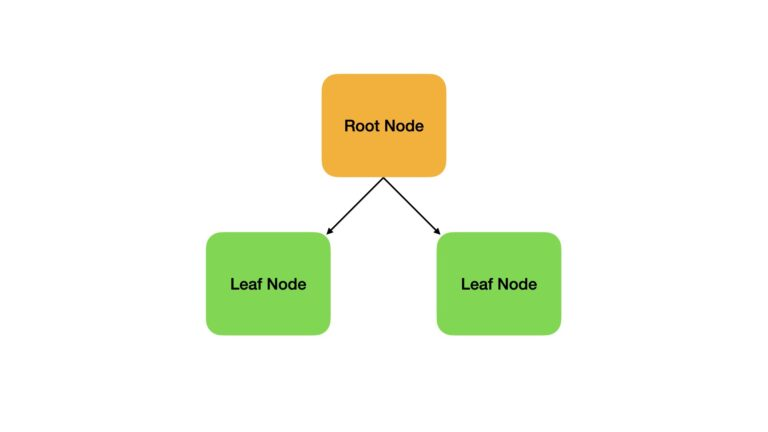We will begin our analysis by importing all the required packages for our work:

## imports ##
import numpy as np
import pandas as pd
from typing import Dict, Any, List, Tuple
from sklearn.base import clone
import matplotlib.pyplot as plt
from sklearn.model_selection import StratifiedKFold,cross_validate
from sklearn.tree import DecisionTreeClassifier
from sklearn.metrics import accuracy_score,precision_score,recall_score,f1_score,make_scorer
from sklearn.ensemble import RandomForestClassifier

### Preliminary Setup

The data we will use here is the Wisconsin Breast Cancer dataset available through scikit-learn. I have already investigated these data in my article on Logistic Regression, and as such I will not repeat that analysis here.

Let’s load these data, and format the labels to adhere to equation (1):

## load classification dataset ##
X    = data.data
y    = data.target

#properly format labels
y = np.where(y==0,-1,1)

We can now define our weak learner decision stump, using the scikit-learn API:

## initialize a weak learner ##
weak_m = DecisionTreeClassifier(max_depth=1)

### Investigate Training Metrics

At this point, I want to check the effects of increasing the ensemble size. Specifically, let’s check that the F1 score of the ensemble increases as more weak learners are added. Conversely, the loss should decrease as more weak learners are added to the ensemble.

We can start by initialising an instance of the Adaboost classifier defined in the previous section. The model will then be trained on the available dataset:

## train the adaboost classifier ##
clf.fit(X,y)

Notice that the record_training_metrics argument is set to True. This tells us that the F1 score and loss were recorded at each iteration during the training procedure.

Let’s obtain the training metrics:

## get training metrics ##
#obtain F1 scores
f1 = clf.get_f1s()
#obtain loss
loss = clf.get_loss()

With the F1 score and loss available, we can now plot these to check the implementation of the training procedure:

## plot the training F1 scores ##
plt.plot(f1)
plt.title('Training F1 score by Number of Component Trees')
plt.xlabel('Number of Component Trees')
plt.ylabel('F1 Score')
plt.show()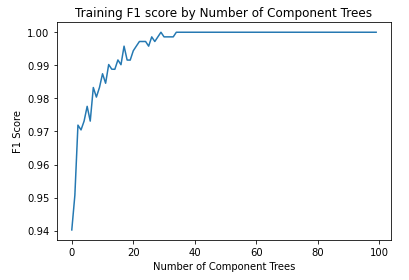## plot the training loss ##
plt.plot(loss)
plt.title('Training Loss by Number of Component Trees')
plt.xlabel('Number of Component Trees')
plt.ylabel('Loss')
plt.show()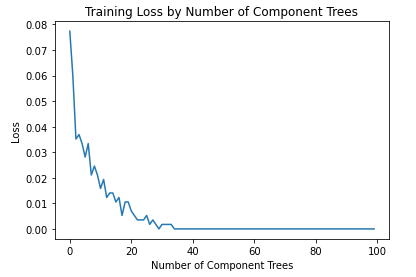The plots indicate our implementation of Adaboost is functioning as one would expect. The F1 score increases, and the loss decreases, as the number of trees included in the ensemble becomes larger. Optimal performance appears to be reached at an ensemble size of approximately 35 trees.

### Cross Validation Analysis

At this point we can look to determine the effectiveness of our model. I will use 10-fold cross-validation to measure the performance of our Adaboost implementation. The metrics included in this analysis will be accuracy, precision, recall, and f1 scores:

## define the scoring metrics ##
scoring_metrics = {'accuracy' : make_scorer(accuracy_score),
'precision': make_scorer(precision_score),
'recall'   : make_scorer(recall_score),
'f1'       : make_scorer(f1_score)}

## perform cross-validation for n_elements=35 ##
#define the model
#cross validate
dcScores = cross_validate(clf,X,y,cv=StratifiedKFold(10),scoring=scoring_metrics)
#report results
print('Mean Accuracy: %.2f' % np.mean(dcScores['test_accuracy']))
print('Mean Precision: %.2f' % np.mean(dcScores['test_precision']))
print('Mean Recall: %.2f' % np.mean(dcScores['test_recall']))
print('Mean F1: %.2f' % np.mean(dcScores['test_f1']))

Mean Accuracy: 0.97

Mean Precision: 0.97

Mean Recall: 0.98

Mean F1: 0.97

These results look quite good, and demonstrate that our Adaboost classifier does a satisfactory job on correctly labelling the available data. Let’s compare these results with the Adaboost model available through scikit-learn:

## import adaboost classifier from scikit-learn ##

## perform cross-validation for n_estimators=35 ##
#define the model
#cross validate
dcScores = cross_validate(clf,X,y,cv=StratifiedKFold(10),scoring=scoring_metrics)
#report results
print('Mean Accuracy: %.2f' % np.mean(dcScores['test_accuracy']))
print('Mean Precision: %.2f' % np.mean(dcScores['test_precision']))
print('Mean Recall: %.2f' % np.mean(dcScores['test_recall']))
print('Mean F1: %.2f' % np.mean(dcScores['test_f1']))

Mean Accuracy: 0.96

Mean Precision: 0.96

Mean Recall: 0.97

Mean F1: 0.96

We can see there is a slight difference between the two Adaboost models, with our custom implementation doing a bit better over all. Note however that the Adaboost classifier available through scikit-learn is not the binary classifier model, but instead follows the SAMME.R implementation. This may explain the small discrepancy between the two Adaboost classifiers.

Now let’s compare our results with a lone decision stump, and a Random Forest Classifier with default hyper parameters:

#cross validate
dcScores = cross_validate(weak_m,X,y,cv=StratifiedKFold(10),scoring=scoring_metrics)
#report results
print('Mean Accuracy: %.2f' % np.mean(dcScores['test_accuracy']))
print('Mean Precision: %.2f' % np.mean(dcScores['test_precision']))
print('Mean Recall: %.2f' % np.mean(dcScores['test_recall']))
print('Mean F1: %.2f' % np.mean(dcScores['test_f1']))

Mean Accuracy: 0.89

Mean Precision: 0.89

Mean Recall: 0.95

Mean F1: 0.91

#declare random forest
clf = RandomForestClassifier()
#cross validate
dcScores = cross_validate(clf,X,y,cv=StratifiedKFold(10),scoring=scoring_metrics)
#report results
print('Mean Accuracy: %.2f' % np.mean(dcScores['test_accuracy']))
print('Mean Precision: %.2f' % np.mean(dcScores['test_precision']))
print('Mean Recall: %.2f' % np.mean(dcScores['test_recall']))
print('Mean F1: %.2f' % np.mean(dcScores['test_f1']))

Mean Accuracy: 0.97

Mean Precision: 0.97

Mean Recall: 0.98

Mean F1: 0.97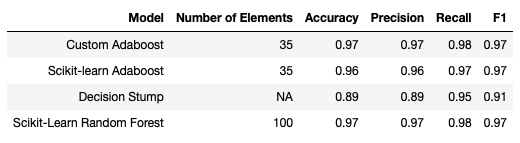Our implementation of the Adaboost algorithm, along with the Random Forest classifier, yields the best overall results from this comparison. However, the scikit-learn Adaboost model performs only marginally worse. Note that the Random Forest Classifier includes 100 individual trees by default. Unsurprising, the lone Decision Stump yields the worst results.

## Final Remarks

• The motivation behind the Adaboost classification algorithm, and when it was developed
• A derivation of the Adaboost algorithm
• How to implement the Adaboost classification algorithm in Python from scratch
• How our implementation of Adaboost compares against open-source, scikit-learn classifier models

I hope you enjoyed this article, and gained some value from it.  If you would like to take a closer look at the code presented here, please take a look at my GitHub. If you have any questions or suggestions, please feel free to add a comment below. Your input is greatly appreciated.

5 1 vote
Article Rating
Subscribe
Notify of1 Comment
Inline Feedbacks